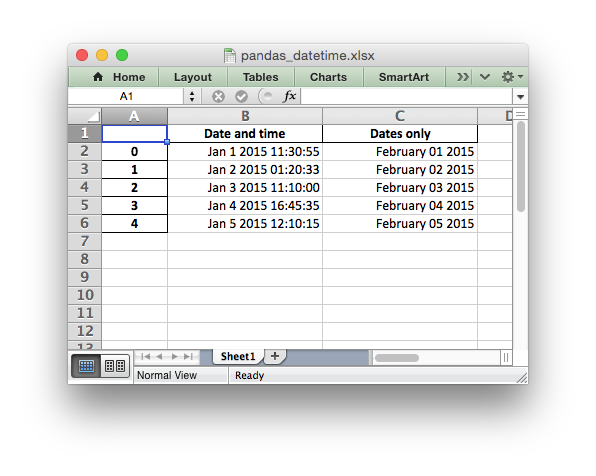# Example: Pandas Excel output with datetimes#

An example of converting a Pandas dataframe with datetimes to an Excel file with a default datetime and date format using Pandas and XlsxWriter.```##############################################################################
#
# An example of converting a Pandas dataframe with datetimes to an xlsx file
# with a default datetime and date format using Pandas and XlsxWriter.
#
# Copyright 2013-2023, John McNamara, jmcnamara@cpan.org
#

import pandas as pd
from datetime import datetime, date

# Create a Pandas dataframe from some datetime data.
df = pd.DataFrame(
{
"Date and time": [
datetime(2015, 1, 1, 11, 30, 55),
datetime(2015, 1, 2, 1, 20, 33),
datetime(2015, 1, 3, 11, 10),
datetime(2015, 1, 4, 16, 45, 35),
datetime(2015, 1, 5, 12, 10, 15),
],
"Dates only": [
date(2015, 2, 1),
date(2015, 2, 2),
date(2015, 2, 3),
date(2015, 2, 4),
date(2015, 2, 5),
],
}
)

# Create a Pandas Excel writer using XlsxWriter as the engine.
# Also set the default datetime and date formats.
writer = pd.ExcelWriter(
"pandas_datetime.xlsx",
engine="xlsxwriter",
datetime_format="mmm d yyyy hh:mm:ss",
date_format="mmmm dd yyyy",
)

# Convert the dataframe to an XlsxWriter Excel object.
df.to_excel(writer, sheet_name="Sheet1")

# Get the xlsxwriter workbook and worksheet objects in order
# to set the column widths and make the dates clearer.
workbook = writer.book
worksheet = writer.sheets["Sheet1"]

# Get the dimensions of the dataframe.
(max_row, max_col) = df.shape

# Set the column widths, to make the dates clearer.
worksheet.set_column(1, max_col, 20)

# Close the Pandas Excel writer and output the Excel file.
writer.close()
```